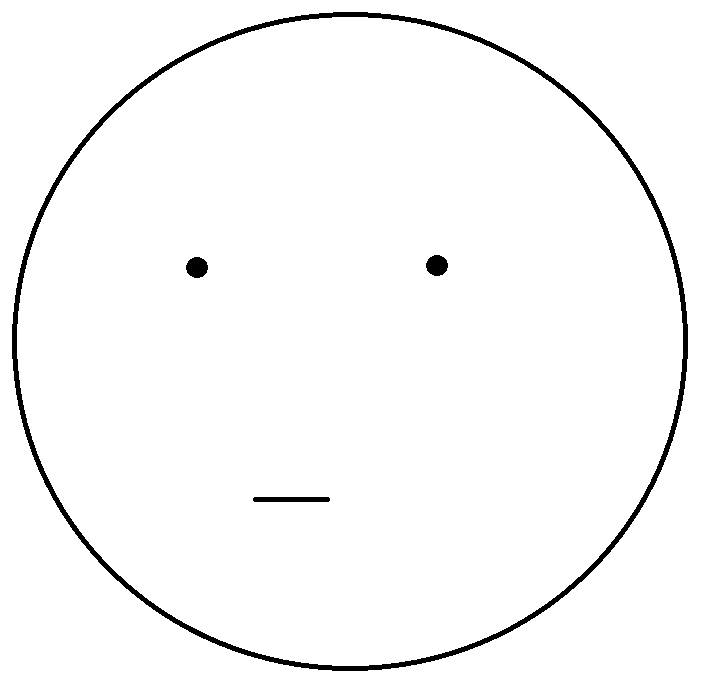# 常用表情符號顏文字精簡版（亞洲、西洋版）OON ME Stupid Face Funny

• 啊哈哈！你看看你！版
• 西洋版
• 亞洲版
• 基本形式
• 複雜形式
• 其他圖示
• 東亞風格
• 傷心
• 生氣
• 道歉
• 無奈、尷尬
• 其他

• (☞ﾟ∀ﾟ)ﾟ∀ﾟ)☞
• σ`∀′) ﾟ∀ﾟ)σ

## 西洋版

• :))　狂笑
• :-)　微笑
• :-(　不悅
• ;-)　使眼色
• :-D　開心
• :-P　吐舌頭
• :-C　很悲傷
• :-O　驚訝、張大口
• :-/　懷疑
• :-S　困惑
• 8-)　戴眼鏡者的微笑
• xc==　嘔
• @–>—-　送你一朵玫瑰花
• <*)>>>=<　魚骨頭
• <□：≡　烏賊
• (：≡　水母
• <※　花束
• XD 大笑
• ：）　笑臉
• ：D 　 笑
• ：ｏ 　驚訝
• =目 超大笑
• :-|　納悶
• <3　心, 愛
• :@ 生氣

## 亞洲版

### 基本形式

• o_O　訝異
• ◎−◎？　疑問
• ^_^　高興
• T_T　哭得很傷心
• =_=”　無奈
• =3=　扭計、嘟嘴
• \$_\$　見錢眼開
• (^_-)db(-_^)　手指打勾勾、約定
• = =b　冒冷汗
• ^_^;;;　冒汗, 不好意思
• ╮(－_－)╭　兩手一攤
• ＝　＝＃　生氣（青筋暴露）
• |(-_-)|　沒聽到（耳朵遮住了）
• /.\　失望
• >< 表示傷心、無奈、嗔怒的廣義表現符號
• ˊˋ因難過而眉毛倒豎
• @_@　暈眩
• = = 無言

### 複雜形式

• (=^_^=)　喵喵
• ((。(^_^)。))　期待、期待
• *\(^_^)/*　拿綵球、為你加油
• ≧◇≦　感動
• (^o^)哈~~(^0^)哈~~(^○^)哈~~　大笑3聲
• (￣﹁￣)　流口水
• ( h_n )　大眼睛和小眼睛
• ‧★,:*:‧\(￣▽￣)/‧:*‧°★*　用力灑花恭喜
• v(￣︶￣)y　得意
• ㄟ(￣▽￣ㄟ)　偷偷逃跑
• ( ￣□￣)/ <(￣ㄧ￣ ) <(￣ㄧ￣ ) 致敬
• (っ´▽`)っ抱抱

### 其他圖示

• ^(00)^　小豬
• = =+
• (˙_˙)/
• >//////< 臉紅(害羞或激動)
• !_!
• @_@
• *_*
• +_+
• @[]@
• ( . 人 . ) 胸部
• vov
• ^Q^
• ~.~
• >3<　kiss
• XD、x)
• o(︶︿︶)o
• @@||
• WoW
• lol 是 laugh out loud 的英文縮寫

### 東亞風格

• ＜（－︿－）＞　我很生氣喔！
• ！別吵我，知道嗎
• >-<　好
• ＜（￣︶￣）＞　滿足且得意，得意快樂
• ＜（￣︶￣）／　滿足並且伸出手，表示贊同、贊成
• ＜（＠￣︶￣＠）＞　滿足地臉紅
• （＃￣▽￣＃）　害羞
• ＼（￣︶￣）／　抱抱、開心
• ｂ（￣▽￣）ｄ　豎起雙手拇指說好
• ╰（￣▽￣）╭　眉飛色舞
• （￣︶￣）↗　出發吧
• ○（＊￣︶￣＊）○　吃飽睡好
• ♀（￣▽￣）／　優質女孩
• ♂（￣▽￣）／　優質男孩
• ╮（╯◇╰）╭　啾～啾～親一個愛你
• ╭（′▽‵）╭（′▽‵）╭（′▽‵）╯　GO!
• （￣▽￣）～■□～（￣▽￣）　乾杯
• ＜（￣︶￣）＞＜（￣︶￣）＞＜（￣︶￣）＞　當我們同在一起
• (*￣▽￣)/‧★*”`’*-.,_,.-*’`”*-.,_☆,.-*`’`*-　流星拳

### 傷心

• （┬＿┬）　流淚
• （＞﹏＜）　痛苦
• （┬＿┬）↘　跌倒
• 〒▽〒　真命苦
• ～＞＿＜～　哭泣中
• T^T”///　飆淚
• >3<　親一個(3表示嘟起的嘴)
• (T_T)　傷心、我哭了
• m(_ _)m 五體投地

### 生氣

• （︶︿︶）　不滿
• ╰（‵□′）╯　暴怒
• （︶︿︶）＝凸　比中指
• （╯－＿－）╯╧╧　翻桌
• （╯‵□′）╯︵┴─┴　翻桌
• ╭∩╮（￣▽￣）╭∩╮　哼，去你的（鄙視你）
• （╬￣皿￣）＝○＃（￣＃）３￣）　看拳
• ＜（‵□′）＞───Ｃε（┬＿┬）３　生氣扭耳朵
• ＜( ￣︿￣)︵θ︵θ︵θ︵θ︵☆（＞口＜－）　無影腳攻擊
• ＝　＝＃　生氣（青筋暴露）
• (#‵′)　哼！
• \(@^０^@)/★　晚安
• (^o^哇)~~(^0^)哈~~(^○^)哈~~　大笑三聲
• 0(^o^)~~YA(^0^)~~SU(^○^)~~MI(^_^) 好好休息（用於睡前、日文）
• <*^.^*>　大笑
• ^+++^ 露齒笑(中間的+越多代表嘴巴開越大，也就是笑的越用力。)
• ^.< 或 >.^ 揪咪、眨眼、打暗號
• =v= 賤賤的笑(中間的嘴有v、V、ˇ等幾種版本。ˇ是注音符號的第三聲，在wiki上打出來似乎因字型會歪掉。)

### 道歉

• 〈（＿　＿）〉　道歉、鞠躬
• ｍ（＿　＿）ｍ　叩拜
• (^〈^)　對不起啦

### 無奈、尷尬

• ╮（￣▽￣）╭
• － －||||
• ┐（─__─）┌
• ╮(︶︿︶)╭
• 0.0 不懂、無辜
• (/＞皿<)/ ～ ┴┴ 翻桌
• (／‵′)／~ ╧╧ 翻桌
• ╯-____-)╯~═╩════╩═~ 翻桌

### 其他

• (￣(工)￣)　大狗熊
• (*> ω <*)超害羞
• ( ▔___▔)y-～　抽菸
• ( ￣ 3￣)y▂ξ　抽菸
• （～￣▽￣～）　裝傻
• ╭(─╴╴─)╮ ▃▇　嗯嗯中，別打擾
• <(￣3￣)>
• －(>口＜-)
• <(‵▽′)>　哈哈哈！我是壞人
• o(〒﹏〒)o　淚
• O-(///￣皿￣)☞ ─═≡☆゜★█▇▆▅▄▃▂＿　佛地魔版之去去武器走
• ( ☉_☉)≡☞o────★°　哈利波特版之去去武器走
• ○(￣︿￣) ○─═★°　榮恩版之去去武器走
• ╮(￣▽￣”)╭　無奈
• ψ(._. )>　筆記
• ≧ω≦　可愛、可愛
• O口O!　超驚訝!
• (*^．^*)　親一個
• (^_-)db(-_^)　手指打勾勾、約定
• ◢▆▅▄▃崩╰(〒皿〒)╯潰▃▄▅▇◣
• \(*O#)3<)▁▂▃▄▅▆▇█ 嗡嗡嗡嗡嗡 －－－－　巫巫茲拉